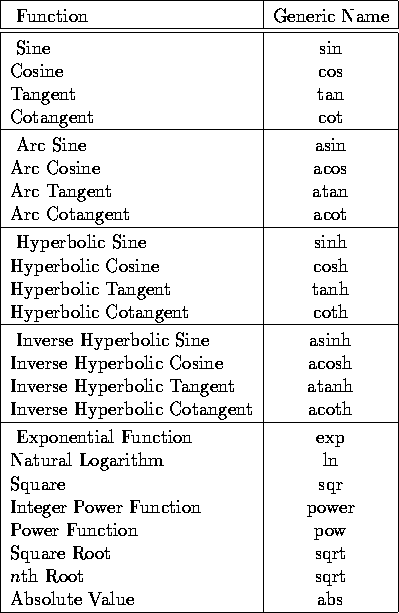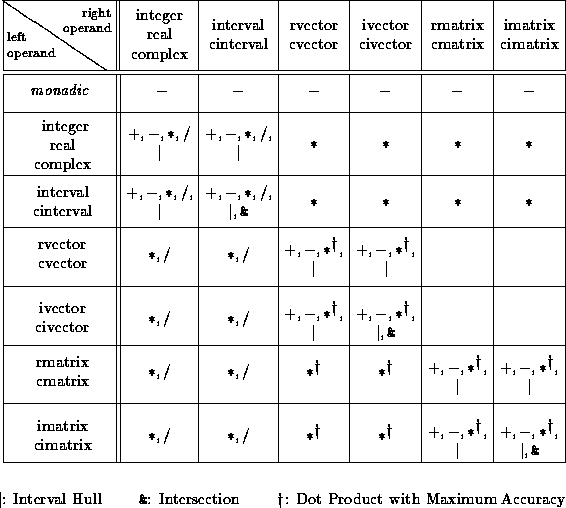Standard Data Types, Predefined Operators, and FunctionsNext: Subarrays of Vectors Up: C-XSC Previous: Introduction

# Standard Data Types, Predefined Operators, and Functions

C-XSC provides the simple numerical data types

real, interval, complex, and cinterval (complex interval)

with their appropriate arithmetic and relational operators and mathematical standard functions. All predefined arithmetic operators deliver results with an accuracy of at least 1 ulp (unit in the last place). Thus, they are of maximum accuracy in the sense of scientific computing. The rounding of the arithmetic operators may be controlled using the data types interval and cinterval. Type casting functions are available for all mathematically useful combinations. Literal constants may be converted with maximum accuracy. All mathematical standard functions for the simple numerical data types may be called by their generic names and deliver results with guaranteed high accuracy for arbitrary permissible arguments. The standard functions for the data types interval and cinterval provide range inclusions which are sharp bounds.Table 1: Mathematical Standard Functions

For the scalar data types presented above, vector and matrix types are available:

rvector, ivector, cvector, and civector,
rmatrix, imatrix, cmatrix, and cimatrix.
The user can allocate or deallocate storage space for a dynamic array (vector or matrix) at run time. Thus, without recompilation, the same program may use arrays of size restricted only by the storage of the computer. Furthermore, the memory is used efficiently, since the arrays are stored only in their required sizes. When accessing components of the array types, the index range is checked at run time to provide increased security during programming by avoiding invalid memory accesses.Table 2: Predefined Arithmetic Operators

Example: Allocation and resizing of dynamic matrices:

```...
int n, m;
cout << "Enter the dimensions n, m:";
cin  >> n >> m;

imatrix B, C, A(n, m);     /* A ... A[n][m] */
Resize(B, m, n);           /* B ... B[m][n] */
...
C = A * B;                 /* C ... C[n][n] */```

Defining a vector or a matrix without explicitly indicating the index bounds results in a vector of length 1 or in a 1x1 matrix. The storage for the object is not allocated until run time. Here, we use the Resize statement (see example above) to allocate an object of the desired size. Alternatively, the index bounds may be determined when defining the vector or matrix as we did in the example above with matrix `A`.

An implicit resizing of a vector or a matrix is also possible during an assignment: If the index bounds of the object on the right-hand side of an assignment do not correspond to those of the left-hand side, the object is changed correspondingly on the left side as shown in the example above with the assignment `C = A * B`.

The storage space of a dynamic array that is local to a subprogram is automatically released before control returns to the calling routine.

The size of a vector or a matrix may be determined at any time by calling the functions Lb() and Ub() for the lower and upper index bounds, respectively.Next: Subarrays of Vectors Up: C-XSC Previous: Introduction

Andreas Wiethoff
Wed Mar 29 13:50:24 MET DST 1995